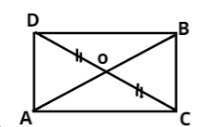Courses
Courses for Kids
Free study material
Free LIVE classes
MoreLIVE
Join Vedantu’s FREE Mastercalss

# In the figure $\vartriangle ABC$ and $\vartriangle ABD$ are two triangles on the same base AB. If line segment CD is bisected by AB at O. Show that $area\left( {\vartriangle ABC} \right) = area\left( {\vartriangle ABD} \right)$Verified
359.4k+ views
Hint: Here we apply the area of triangle theorem where median divides the triangle in two equal areas.

In $\vartriangle ABC$
AO is the median, (CD is bisected by AB at O)
As you know, the median divides the triangle in two equal areas.
So,$area\left( {\vartriangle AOC} \right) = area\left( {\vartriangle AOD} \right)$ …… (1)
Also, in $\vartriangle BCD$
BO is the median, (CD is bisected by AB at O)
So, $area\left( {\vartriangle BOC} \right) = area\left( {\vartriangle BOD} \right)$ …… (2)
$area\left( {\vartriangle AOC} \right) + area\left( {\vartriangle BOC} \right) = area\left( {\vartriangle AOD} \right) = area\left( {\vartriangle BOD} \right)$
$\Rightarrow area\left( {\vartriangle ABC} \right) = area\left( {\vartriangle ABD} \right)$